## 70. 爬楼梯

You are climbing a stair case. It takes n steps to reach to the top.

Each time you can either climb 1 or 2 steps. In how many distinct ways can you climb to the top?

Note: Given n will be a positive integer.

Example 1:

Example 2:

Example 1:

Example 2:

## 64. 最小路径和

dp[i] [j] 为每个格子到`左上角`最短路径 ，每个格子到左上角的最小距离等于，左边格子最小值和上边格子最小值的最小值加上当前格子的值。

UPDATE: 2020.7.20

## Path sum: three ways

The minimal path sum in the 5 by 5 matrix below, by starting in any cell in the left column and finishing in any cell in the right column, and only moving up, down, and right, is indicated in red and bold; the sum is equal to 994.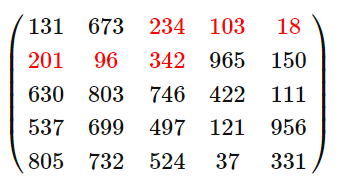Find the minimal path sum from the left column to the right column in matrix.txt (right click and “Save Link/Target As…”), a 31K text file containing an 80 by 80 matrix.

## 62. 不同路径## 63. 不同路径 IIUPDATE: 2020.7.6

## 576. 出界的路径数

1. 球一旦出界，就不能再被移动回网格内。
2. 网格的长度和高度在 [1,50] 的范围内。
3. N 在 [0,50] 的范围内。

K 放在最外层的原因其实很容易想到，我们求某一个点的时候，我们需要周围 4 个方向的`K-1`的值，这些值必须是已经计算过的，所以 K 肯定是要放在最外层的

## 688. “马”在棋盘上的概率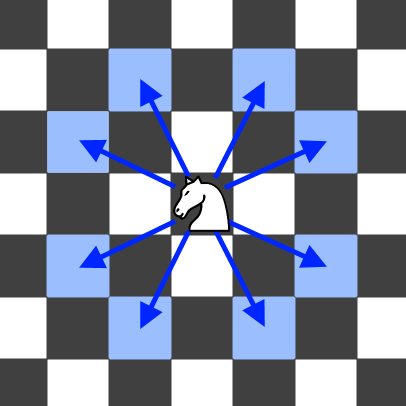• `N` 的取值范围为 [1, 25]
• `K` 的取值范围为 [0, 100]
• 开始时，“马” 总是位于棋盘上

## 935. 骑士拨号器.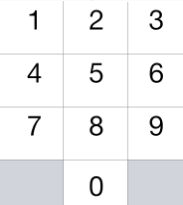• `1 <= N <= 5000`

## 303. 区域和检索 - 数组不可变

• 你可以假设数组不可变。
• 会多次调用 sumRange 方法。

## 413. 等差数列划分

dp 的题啊，答案一看就明白，就是自己想不到。

## 343. 整数拆分`dp[i] = max(dp[i]，max(dp[ i-j ] * j ，(i-j) * j));`

## 279. 完全平方数

`dp[i] =min(dp[i]，dp[i-j*j]+1)`

50%左右，看了下时间复杂度应该是 `O(N^1.5)` 还是挺高的（评论区用 Py 的都超时了）

BFS，将数字按照完全平方数连接为一个图，然后 BFS 求一个从 n 到 0 的最短的路径

## 91. 解码方法

80%左右，这题其实一开始并不是这样做的，我觉得我开始的思路还是挺好的，将字符串分段，当前一个和后一个无法合并的时候就作为一个分界点，最后将每一段的编码方式相乘就是结果了。

💬 `s_num[i-1]!=0 && s_num[i-2]!=0 && s_num[i-2]+s_num[i-1] <=26`

💬 `s_num[i-1]==0 && s_num[i-2]!=0 && s_num[i-2]+s_num[i-1] <=26`

💬 `s_num[i-1]!=0 && s_num[i-2]!=0 && s_num[i-2]+s_num[i-1] >26`

💬 `s_num[i-1]!=0 && s_num[i-2]==0 && s_num[i-2]+s_num[i-1] <=26`

💬 `s_num[i-1]==0 && s_num[i-2]==0` 或者`s_num[i-1]==0 && s_num[i-2]!=0 && s_num[i-2]+s_num[i-1]>26`

## 面试题 46. 把数字翻译成字符串

• `0 <= num < 231`

## 5375. 恢复数组

• 1 <= s.length <= 10^5.
• s 只包含数字且不包含前导 0 。
• 1 <= k <= 10^9.

24th 双周赛的 T4，其实 dp 的状态转换还是想出来了，我想的是从左向右，但是细节没理清楚，瞄了一眼评论区，发现从右往左比较简单

## 300. 最长上升子序列

• 可能会有多种最长上升子序列的组合，你只需要输出对应的长度即可。
• 你算法的时间复杂度应该为 O(n2) 。
• 进阶：你能将算法的时间复杂度降低到 O(n log n) 吗？

LIS，这题好像在很多地方见到过了，还是要好好理解下，dp[i] 表示以当前元素结尾的最长上升子序列的长度`贪心+二分` 这个贪心还是有点骚的，核心思想：

2020.3.20（copy 自从 liweiwei 大佬 题解

## 1016. 使序列递增的最小交换次数（LintCode）

• A, B 是长度相同的数组，它们的长度范围为 [1, 1000]。
• A[i], B[i] 是在 [0, 2000] 范围内的整数。

## 354. 俄罗斯套娃信封问题

2020.3.20 阿里笔试考了这一题，dp 超时了。

UPDATE: 2020.7.13

## 646. 最长数对链

• 给出数对的个数在 [1, 1000] 范围内。

`每次都在列表中找第二个元素最小的数对依次组成链，最后得到的一定是最长的链`

1. 全在左边
2. 全在右边
3. 跨中点，两边都有

## 376. 摆动序列

• 你能否用 O(n) 时间复杂度完成此题？

O(N^2 ) 动态规划，dp[i] 代表目前为止以 nums[i] 结尾的最长的`上升摇摆序列`，down[i] 代表目前为止以 nums[i] 结尾的最长的`下降摇摆序列`，后面过程就和上面的`LIS`的解法类似了

## 978. 最长湍流子数组

`A` 的子数组 `A[i], A[i+1], ..., A[j]` 满足下列条件时，我们称其为湍流子数组

• `i <= k < j`，当 `k` 为奇数时， `A[k] > A[k+1]`，且当 `k` 为偶数时，`A[k] < A[k+1]`
• `i <= k < j`，当 `k` 为偶数时，`A[k] > A[k+1]` ，且当 `k` 为奇数时， `A[k] < A[k+1]`

1. `1 <= A.length <= 40000`
2. `0 <= A[i] <= 10^9`

## 96. 不同的二叉搜索树

Given n, how many structurally unique BST’s (binary search trees) that store values 1 … n?

Example:

`i` 位置元素为根节点，可以得出可能的 BST 数量为，`[1 ~ i-1]` 可以构成的 BST 数量 乘以 `[i+1，n]`可以构成的 BST 数量，也就是左子树 * 右子树 ，再推广到整个序列的 BST 总数，其实也就是 以每个元素为根节点构成的 BST 的数量之和 ，需要理解的地方就是`dp[i]` 的含义，明确本题中`dp[i]` 其实只和长度 `i`有关而和内容无关，比如 1，2，3 和 4，5，6 所能构成的 BST 数量其实是相同的

## 120. 三角形最小路径和

O(N) 空间复杂度，只和下面那一层的每一个元素的 dp[i] 有关，所以可以直接改成一维 dp

UPDATE: 2020.7.14

## 122. 买卖股票的最佳时机 IIA->D 是一段连续上涨日，`D-A 的差值` 就是 A->D 各个相邻节点差值之和## 714. 买卖股票的最佳时机含手续费

• `0 < prices.length <= 50000.`
• `0 < prices[i] < 50000.`
• `0 <= fee < 50000.`

## 309. 最佳买卖股票时机含冷冻期

• 你不能同时参与多笔交易（你必须在再次购买前出售掉之前的股票）
• 卖出股票后，你无法在第二天买入股票 （即冷冻期为 1 天）`dp[i] = max(dp[i-1], dp[i-1] + prices[i])`

`dp[i] = max(dp[i-1], dp[i-1] - prices[i])`

`dp[i-1] = max(dp[i-2], dp[i-2] + prices[i-1])`

`dp[i] = max(dp[i-1], max(dp[i-2], dp[i-2] + prices[i-1]) - prices[i])`

`dp[i] = max(dp[i-1], max(dp[i-2]) - prices[i])`

## 1269. 停在原地的方案数

• `1 <= steps <= 500`
• `1 <= arrLen <= 10^6`

11.24 的周赛最后一题，很可惜没做出来。要是把这题放在第三题我可能就做出来了。还是菜啊

## 152. 乘积最大子序列

`max[i],min[i]` 代表的就是以`nums[i]` 结尾的最大乘积和最小乘积

• `nums[i] >= 0` 并且`max[i-1] > 0``max[i] = max[i-1] * nums[i]`

• `nums[i] >= 0` 并且`max[i-1] < 0`，此时如果和前边的数累乘的话，会变成负数，所以`max[i] = nums[i]`

• `nums[i] < 0`，如果是负数就不应该再和前面`max[i]`相乘，而是考虑`min[i]`

• `当 min[i-1] < 0，max[i] = min[i-1] * nums[i]`
• `当 min[i-1] >= 0，max[i] = nums[i]`

然后我们直接求这三种情况的最大值就 ok 了，不用考虑那些分支，我上面的第一种解法其实就是考虑了所有分支，结果才写出了那样的 dp， `min[i]` 的过程和上面一样，就不赘述

## 1567. 乘积为正数的最长子数组长度

Difficulty: 中等

• `1 <= nums.length <= 10^5`
• `-10^9 <= nums[i] <= 10^9`

## 1594. 矩阵的最大非负积

Difficulty: 中等

• `1 <= rows, cols <= 15`
• `-4 <= grid[i][j] <= 4`

## 1277. 统计全为 1 的正方形子矩阵

• `1 <= arr.length <= 300`
• `1 <= arr.length <= 300`
• `0 <= arr[i][j] <= 1`

12.1 的周赛题目，其实先做的上面的最大正方形，然后发现这一题和上面的一样。

## 1139. 最大的以 1 为边界的正方形

Difficulty: 中等

• `1 <= grid.length <= 100`
• `1 <= grid.length <= 100`
• `grid[i][j]` 为 `0` 或 `1`（之前的图有点小问题，改了下）

## 44. 通配符匹配

• s 可能为空，且只包含从 a-z 的小写字母。
• p 可能为空，且只包含从 a-z 的小写字母，以及字符 ? 和 *。

## 10. 正则表达式匹配

• s 可能为空，且只包含从 a-z 的小写字母。
• p 可能为空，且只包含从 a-z 的小写字母，以及字符 . 和 *。

dfs 回溯，暴力解法，挺难搞

## 1326. 灌溉花园的最少水龙头数目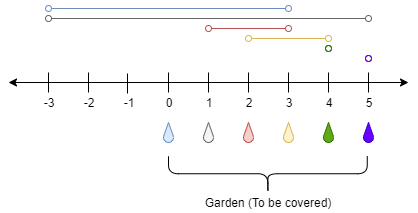• `1 <= n <= 10^4`
• `ranges.length == n + 1`
• `0 <= ranges[i] <= 100`

172 周赛的最后一题

## 1335. 工作计划的最低难度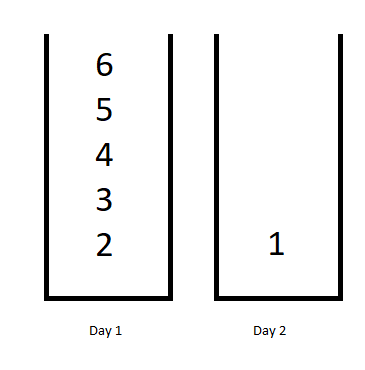173 周赛的最后一道 dp 题，事后一个小时左右独立的 ac，还是挺舒服的，希望以后能更快 ac

`dp[i][j]` 的含义是前`i`天完成前`j`项工作的最低难度，最后返回的结果就是`dp[d-1][jlen-1]`

## 5331. 跳跃游戏 V

• `i + x` ，其中 `i + x < arr.length 且 0 < x <= d`
• `i - x` ，其中 `i - x >= 0 且 0 < x <= d`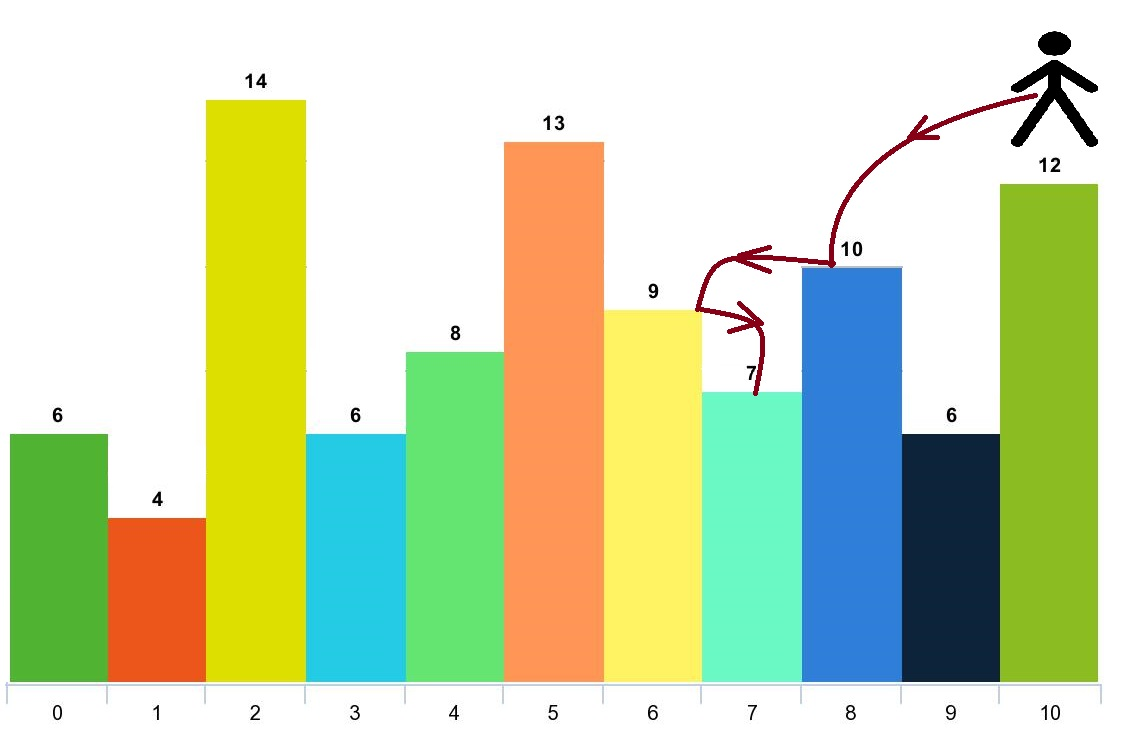• `1 <= arr.length <= 1000`
• `1 <= arr[i] <= 10^5`
• `1 <= d <= arr.length`

## 1262. 可被三整除的最大和

• `1 <= nums.length <= 4 * 10^4`
• `1 <= nums[i] <= 10^4`

dp 边界的处理方式还是要多练啊

## 面试题 60. n 个骰子的点数

`1 <= n <= 11`

`dp[i][j]`代表** i 枚色子和为 j **的概率 递推公式很容易想到 `dp[i][j]= 1/6(dp[i-1][j-1]+dp[i-1][j-2]+dp[i-1][j-3]...dp[i-1][j-6])` 然后我们枚举各个状态就 ok 了

## 264. 丑数 II

1. 是丑数。
2. n 不超过 1690。

`HashSet`去重

## 面试题 62. 圆圈中最后剩下的数字

0,1,,n-1 这 n 个数字排成一个圆圈，从数字 0 开始，每次从这个圆圈里删除第 m 个数字。求出这个圆圈里剩下的最后一个数字。

• 1 <= n <= 10^5
• 1 <= m <= 10^6

## 1014. 最佳观光组合

• `2 <= A.length <= 50000`
• `1 <= A[i] <= 1000`

## 983. 最低票价

• 一张为期一天的通行证售价为 `costs` 美元；
• 一张为期七天的通行证售价为 `costs` 美元；
• 一张为期三十天的通行证售价为 `costs` 美元。

1. `1 <= days.length <= 365`
2. `1 <= days[i] <= 365`
3. `days` 按顺序严格递增
4. `costs.length == 3`
5. `1 <= costs[i] <= 1000`

## 837. 新 21 点

1. `0 <= K <= N <= 10000`
2. `1 <= W <= 10000`
3. 如果答案与正确答案的误差不超过 `10^-5`，则该答案将被视为正确答案通过。
4. 此问题的判断限制时间已经减少。

Difficulty: 困难

## 1143. 最长公共子序列

2019.10.19 力扣终于有这题了，重新来做一遍，这题也就是经常说的 LCS，dp 的经典问题

• `dp[i][j]` 代表 A 序列前`i`个字符（记为`Ai`）， 和 B 序列前`j`个字符（记为`Bj`） 的 LCS

• 两层 for 循环遍历两个序列，当`A[i]==B[j]`的时候，LCS 长度就会增加 1，进而得到 dp 方程

`dp[i][j]=dp[i-1][j-1]+1` dp[i-1] [j-1] 代表的就是不包含`Ai/Bj``Ai-1``Bj-1` 的 LCS

• `A[i]!=B[j]`的时候就有两种情况了，首先`A[i]``B[j]`已经不可能同时出现在最终的 LCS 里面了，所以我们考虑另外的两种情况`dp[i][j-1]``dp[i-1][j]`取个最大值

2019.10.19 重写了一种记忆化递归的方法，没有 dp 快，但是思路其实是类似的

## 718. 最长重复子数组

1. 1 <= len(A), len(B) <= 1000
2. 0 <= A[i], B[i] < 100

_已补充_，见 Rabin-Karp 专题

## 72. 编辑距离

• 插入一个字符
• 删除一个字符
• 替换一个字符

`minDis(i,j)=minDis(i-1,j-1) word1[i]==word2[j]`

`minDis(i,j)=min(minDis(i-1,j),minDis(i,j-1),minDis(i-1,j-1))+1 word1[i]!=word2[j]`

## 647. 回文子串

1. 输入的字符串长度不会超过 1000。

## 32. 最长有效括号

Update

Update: 2020.7.4

UPDATE: 2020.7.4，把之前所谓的“神奇”的方法也记录下，其实也没啥神奇的，看一下就懂了，这种方法还是很优秀的，空间复杂度为`O(1)`

## 面试题 17.13. 恢复空格

Difficulty: 中等

• `0 <= len(sentence) <= 1000`
• `dictionary`中总字符数不超过 150000。
• 你可以认为`dictionary``sentence`中只包含小写字母。

## 174. 地下城游戏

Difficulty: 困难

 -2 (K) -3 3 -5 -10 1 10 30 -5 (P)

• 骑士的健康点数没有上限。

• 任何房间都可能对骑士的健康点数造成威胁，也可能增加骑士的健康点数，包括骑士进入的左上角房间以及公主被监禁的右下角房间。

## 97. 交错字符串

Difficulty: 困难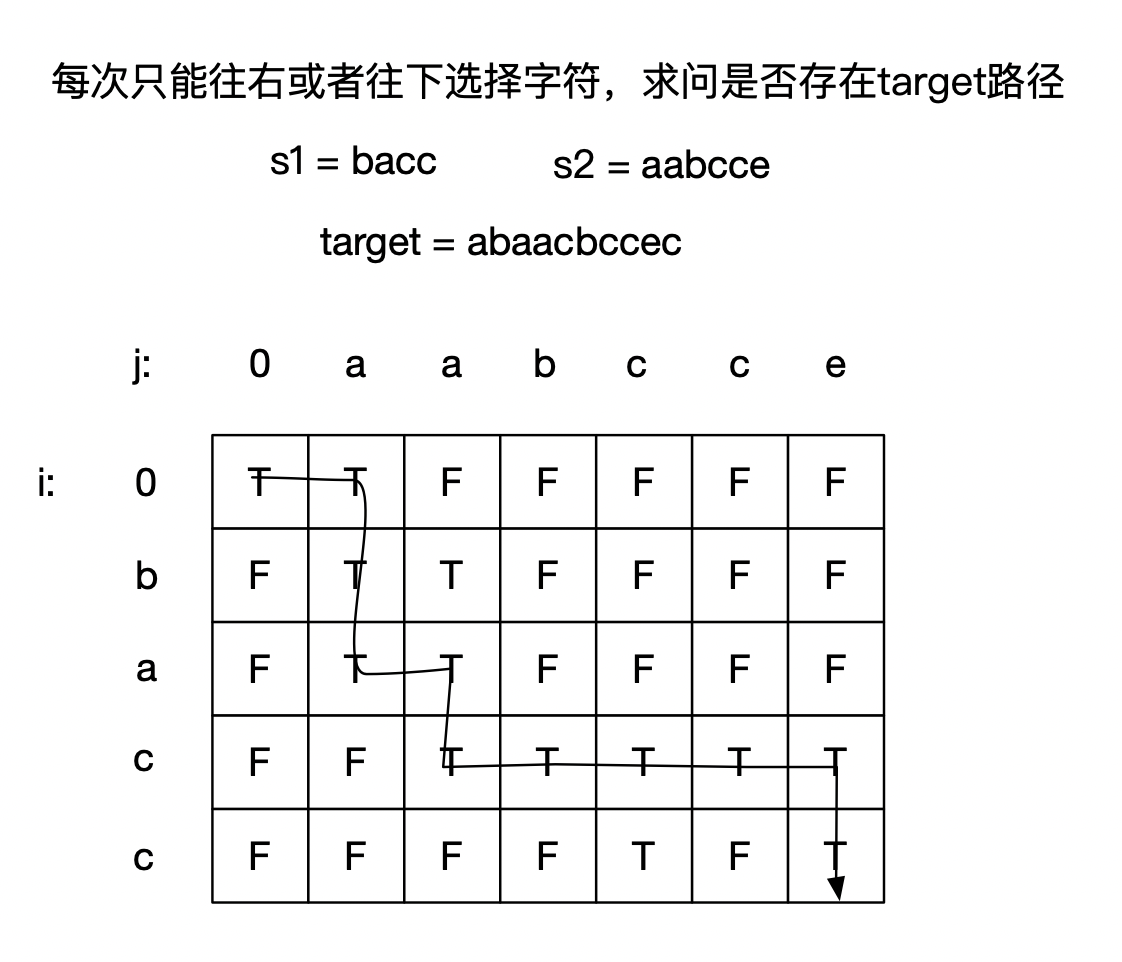## 857. 最小的窗口子序列（LintCode）

1. 输入中的所有字符串只包含小写字母。
2. S 的长度范围在 [1, 20000]。
3. T 的长度范围在 [1, 100]。

LeetCode 上是会员题，所以在 LintCode 上写的，一开始以为是滑窗，想了半天，也只有暴力的思路，看了下讨论区，发现大家都是 dp 做的。

## 1553. 吃掉 N 个橘子的最少天数

Difficulty: 困难

• 吃掉一个橘子。
• 如果剩余橘子数 `n` 能被 2 整除，那么你可以吃掉 `n/2` 个橘子。
• 如果剩余橘子数 `n` 能被 3 整除，那么你可以吃掉 `2*(n/3)` 个橘子。

• `1 <= n <= 2*10^9`

## NC549. 变向

1. 不花费金币跑到第 i 行第 j+1 列
2. 花费`m[j]`的金币跑到第 i-1 行第 j+1 列（如果 i=1 则不可以这么跑）。
3. 花费`m[j]`的金币跑到第 i+1 行第 j+1 列（如果 i=3 则不可以这么跑）。
（牛牛是一个富豪，本身带了很多的金币，所以你不用担心他钱不够用）
现在告诉你所有格子的金币数量和每一列的金币花费，牛牛想知道他跑到第 n 列最多可以赚得多少金币（赚得金币=获得金币-消耗金币）

• 2 <= n <= 1e5

• 第 1 个参数 n 代表跑道的列数
第 2，3，4 个参数 vector a1,a2,a3 各有 n 个元素，代表第 1，2，3 行每一列的金币个数
第 5 个参数 vector m 有 n 个元素代表每一列进行换行的时候需要的金币花费

## NC554 简单变向

1. 跑到第 i 行第 j+1 列
2. 跑到第 i-1 行第 j+1 列（如果 i=1 则不可以这么跑）。
3. 跑到第 i+1 行第 j+1 列（如果 i=3 则不可以这么跑）。

## 787. K 站中转内最便宜的航班

Difficulty: 中等

`n` 个城市通过 `m` 个航班连接。每个航班都从城市 `u` 开始，以价格 `w` 抵达 `v`

• `n` 范围是 `[1, 100]`，城市标签从 `0``n` `- 1`.
• 航班数量范围是 `[0, n * (n - 1) / 2]`.
• 每个航班的格式 `(src,` `dst``, price)`.
• 每个航班的价格范围是 `[1, 10000]`.
• `k` 范围是 `[0, n - 1]`.
• 航班没有重复，且不存在环路

## NC557. 好多牛牛

10 <= len(s) <= 1e5

## 926. 将字符串翻转到单调递增

Difficulty: 中等

1. `1 <= S.length <= 20000`
2. `S` 中只包含字符 `'0'` 和 `'1'`

## 845. 数组中的最长山脉

Difficulty: 中等

• `B.length >= 3`
• 存在 `0 < i < B.length - 1` 使得 `B < B < ... B[i-1] < B[i] > B[i+1] > ... > B[B.length - 1]`

（注意：B 可以是 A 的任意子数组，包括整个数组 A。）

1. `0 <= A.length <= 10000`
2. `0 <= A[i] <= 10000`

## 312. 戳气球

• 你可以假设 nums[-1] = nums[n] = 1，但注意它们不是真实存在的所以并不能被戳破。
• 0 ≤ n ≤ 500, 0 ≤ nums[i] ≤ 100

`dp[i][j]=max(coins[k]* coins[k-1] * coins[k+1]+dp[i][k-1]+dp[k+1][j]) k∈[i,j]`,

`dp[i][j]=max(coins[k]*coins[i-1]*coins[j+1]+dp[i][k-1]+dp[k+1][j]) k∈[i,j]`

UPDATE:2020.7.19

## 877. 石子游戏

1. `2 <= piles.length <= 500`
2. `piles.length` 是偶数。
3. `1 <= piles[i] <= 500`
4. `sum(piles)` 是奇数。

## 1039. 多边形三角剖分的最低得分## 516. 最长回文子序列

1. `s[i]==p[j]``dp[i][j]=dp[i+1][j-1]+2`
2. `s[i]!=p[j]``dp[i][j]=max(dp[i+1][j],dp[i][j-1])`

UPDATE

## 1312. 让字符串成为回文串的最少插入次数

「回文串」是正读和反读都相同的字符串。

• 1 <= s.length <= 500
• s 中所有字符都是小写字母。

170 周赛的压轴题，其实和前面的 516. 最长回文子序列是一样的。没啥好说的

UPDATE：2020.5.18，区间 dp 的写法

## 664. 奇怪的打印机

`dp[left][right]`代表 left 到 right 的最少打印次数，然后我们要理解一点：`dp[left][right]` 其实只可能有两个取值，`dp[left][right-1]`或者`dp[left][right-1]+1`，我们求最小打印次数其实就是希望增加一个字符后打印次数不变

eg: abc b 这个最后的 b 就可以连同前面的 bc 一起顺带打印出来，打印次数不变

`dp[left][right]=dp[left][i-1]+dp[i][right-1]`

ps: 这几天做了不少有点难度的 DP 题，发现确实自己对边界的处理，递推公式的理解有了一点提升，加油💪

## 1547. 切棍子的最小成本

Difficulty: 困难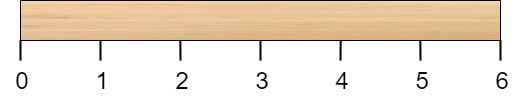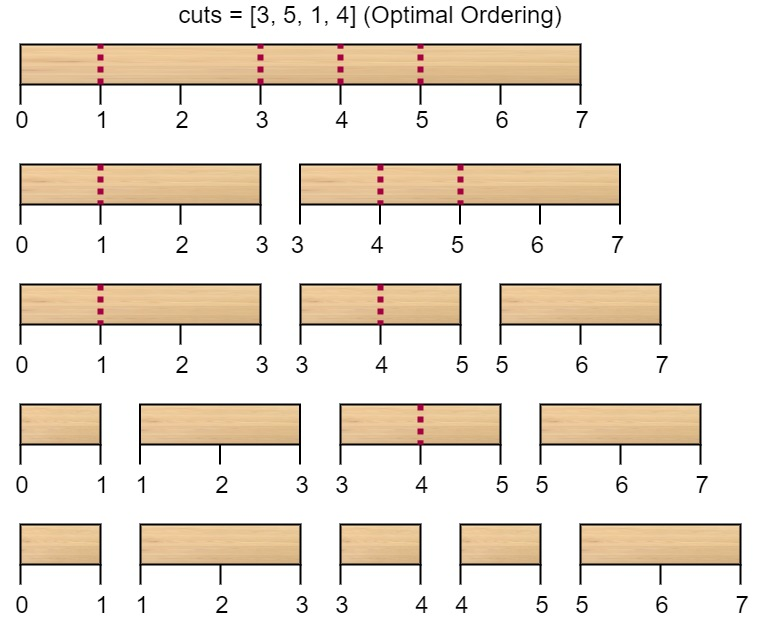• `2 <= n <= 10^6`
• `1 <= cuts.length <= min(n - 1, 100)`
• `1 <= cuts[i] <= n - 1`
• `cuts` 数组中的所有整数都 互不相同

201th 周赛的 t4，感觉还是挺简单的，今天补的时候花了 10 分钟搞清楚思路，一遍看视频一边写，最后不出意外的的 T 了~😂一开始没想清楚细节，想到了以区间开始和结尾做 dp，然后就直接开了个 n*n 的数组，虽然是对的，但是在初始化的时候就 t 了，这里实际上只需要开一个 len(cuts)^2 的空间就行了

## 233. 数字 1 的个数

Update: 2020.6.24

## 1420. 生成数组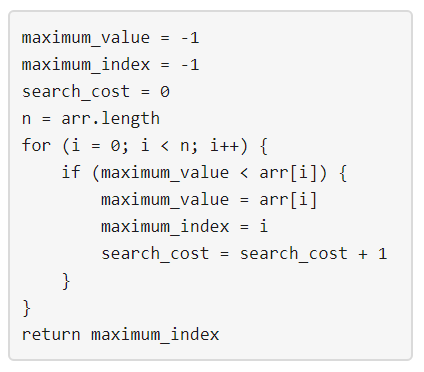• arr 中有 n 个整数。
• 1 <= arr[i] <= m 其中 (0 <= i < n) 。
• 将上面提到的算法应用于 arr ，search_cost 的值等于 k 。

• 1 <= n <= 50
• 1 <= m <= 100
• 0 <= k <= n

185 周赛 T4，类似于数位 DP，记忆化递归的方式很好理解

## 902. 最大为 N 的数字组合

Difficulty: 困难

1. `D` 是按排序顺序的数字 `'1'-'9'` 的子集。
2. `1 <= N <= 10^9`

lc 上写的第 3 道数位 DP，套模板，理解的还不够深刻，等以后做多了再单独开专题讲解

## 1025. 除数博弈

Difficulty: 简单

• 选出任一 `x`，满足 `0 < x < N` 且 `N % x == 0` 。
• `N - x` 替换黑板上的数字 `N`

1. `1 <= N <= 1000`

## 1140. 石子游戏 II

Difficulty: 中等

• `1 <= piles.length <= 100`
• `1 <= piles[i] <= 10 ^ 4`

## 1406. 石子游戏 III

Difficulty: 困难

Alice 和 Bob 用几堆石子在做游戏。几堆石子排成一行，每堆石子都对应一个得分，由数组 `stoneValue` 给出。

Alice 和 Bob 轮流取石子，Alice 总是先开始。在每个玩家的回合中，该玩家可以拿走剩下石子中的的前 1、2 或 3 堆石子 。比赛一直持续到所有石头都被拿走。

• `1 <= values.length <= 50000`
• `-1000 <= values[i] <= 1000`

## 1510. 石子游戏 IV

Difficulty: 困难

Alice 和 Bob 两个人轮流玩一个游戏，Alice 先手。

• `1 <= n <= 10^5`

## 486. 预测赢家

Difficulty: 中等

• 1 <= 给定的数组长度 <= 20.
• 数组里所有分数都为非负数且不会大于 10000000 。
• 如果最终两个玩家的分数相等，那么玩家 1 仍为赢家。

## 743. 网络延迟时间

`N` 个网络节点，标记为 `1``N`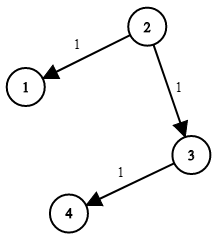1. `N` 的范围在 `[1, 100]` 之间。
2. `K` 的范围在 `[1, N]` 之间。
3. `times` 的长度在 `[1, 6000]` 之间。
4. 所有的边 `times[i] = (u, v, w)` 都有 `1 <= u, v <= N``0 <= w <= 100`

## 1584. 连接所有点的最小费用

Difficulty: 中等• 1 <= points.length <= 1000
• -106 <= xi, yi <= 106
• 所有点 (xi, yi) 两两不同。

Prim O(V^2) 最小生成树，206th 周赛 t3，裸的 mst，但是彩笔的我并不会，事后补了一波 OI Wiki，（这个是原始的暴力 Prim，应对这个题够了）

Kruskal + 并查集，比较适合稀疏图，这里时机复杂度是 O(ElogE)，E 为边数